# Printable Ten FramesFeb 22, 2015FrameBy PrintableeNo CommentPrintable Ten Frames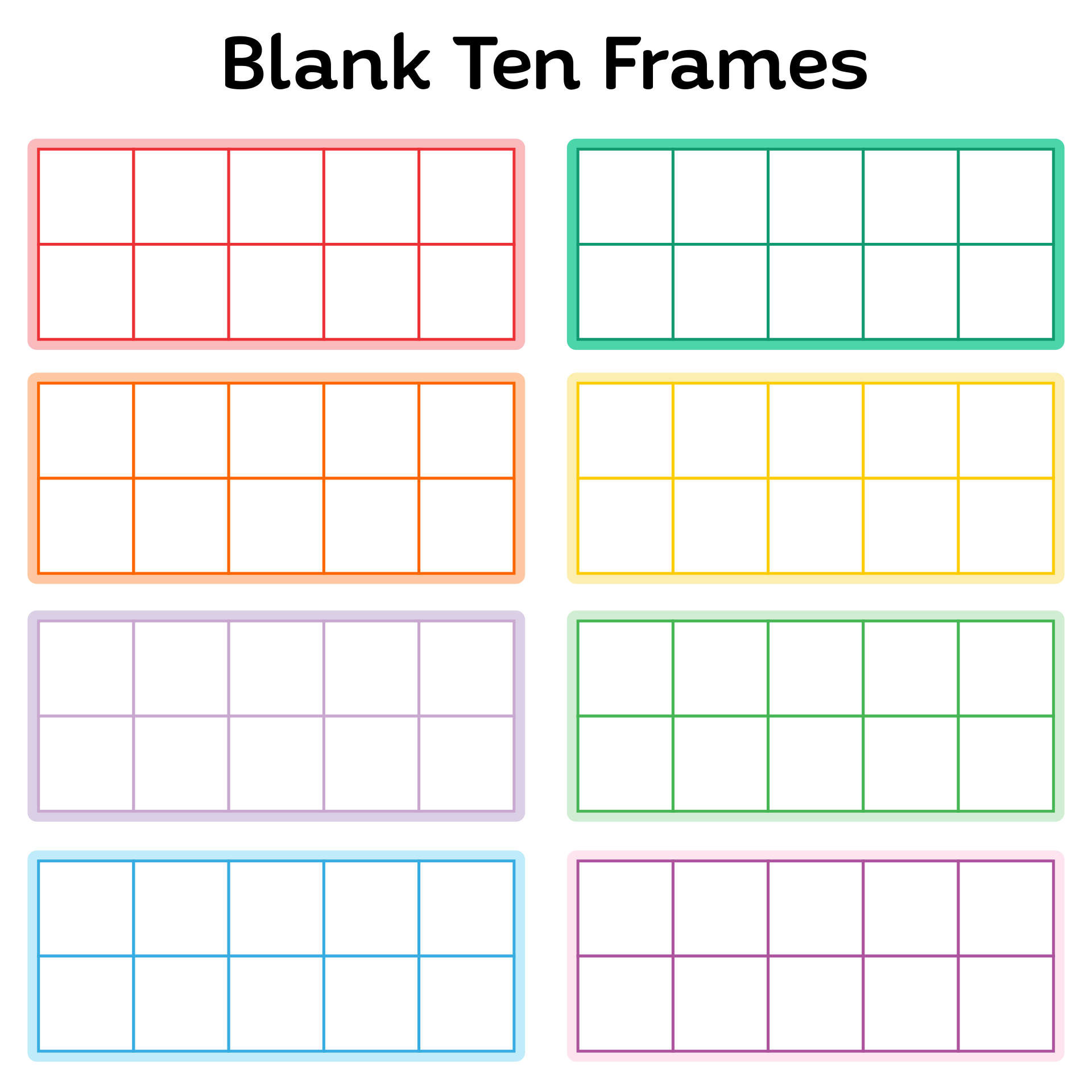### What is the 10 frame?

Not only number lines and number charts, but the ten frames are also one of the helpful tools for the kids. Before we download ten frames and use it for learning math with kids, we are required to understand what ten frames are.

Well, the ten frames can be defined as a grid that consists of two by five boxes or cells. Each cell of the frames is commonly filled by a circle.

These ten frames help the kids to learn about the concept of less than, more than, or equal to a certain number. In this case, the benchmark number is number 10.

### How do ten frames help students?

There must be certain skills that are improved when it comes to using this kind of tool for learning math for kids, the ten frames. Actually, the ten frames allow the children to improve their sensing skills, specifically number sensing skills.

In these ten frames, besides the number 10, number 5 is also used as a benchmark. The ten frames is a good tool to help them visualize the concept of each number.
For instance, if we fill 2 out of 10 frames, then they are required to answer quickly the number of the blank cells and the filled cells. Yes, there are no numbers required. This is because we want to develop visualizing number skills.

Then, after that, we can ask the kids, which one has the higher number, the blank cells, or the filled cells. We can also test them how many cells required to make all the grid is completely filled. So, yes. This is good to improve number sensing skills and visualize the concept of mathematical numbers.

### What is number sense math?

Yes, one of the skills that can be trained using ten frames for learning math is to develop the number sense skills. However, before we download ten frames worksheet and use it for our kids, we are required to understand what number sense skills are.

Actually, number sense can be defined as a qualification of many kinds of mathematical abilities. When it comes to number sense skills, which will be improved when using ten frames, this number sense skills involve the capability to know and analyze the amount (quantities) and the concept of the comparison, like less than, more than, or equals to a certain number.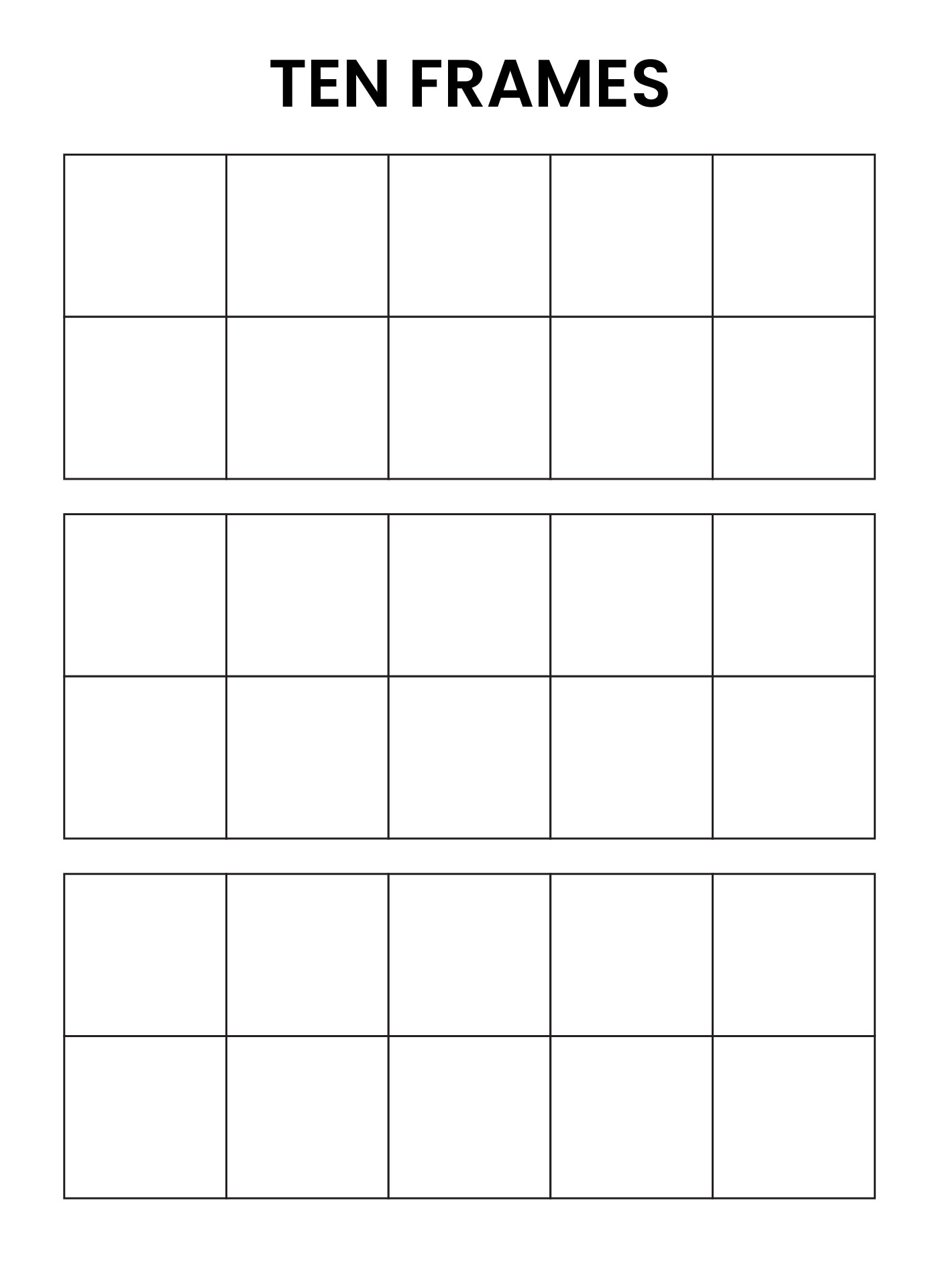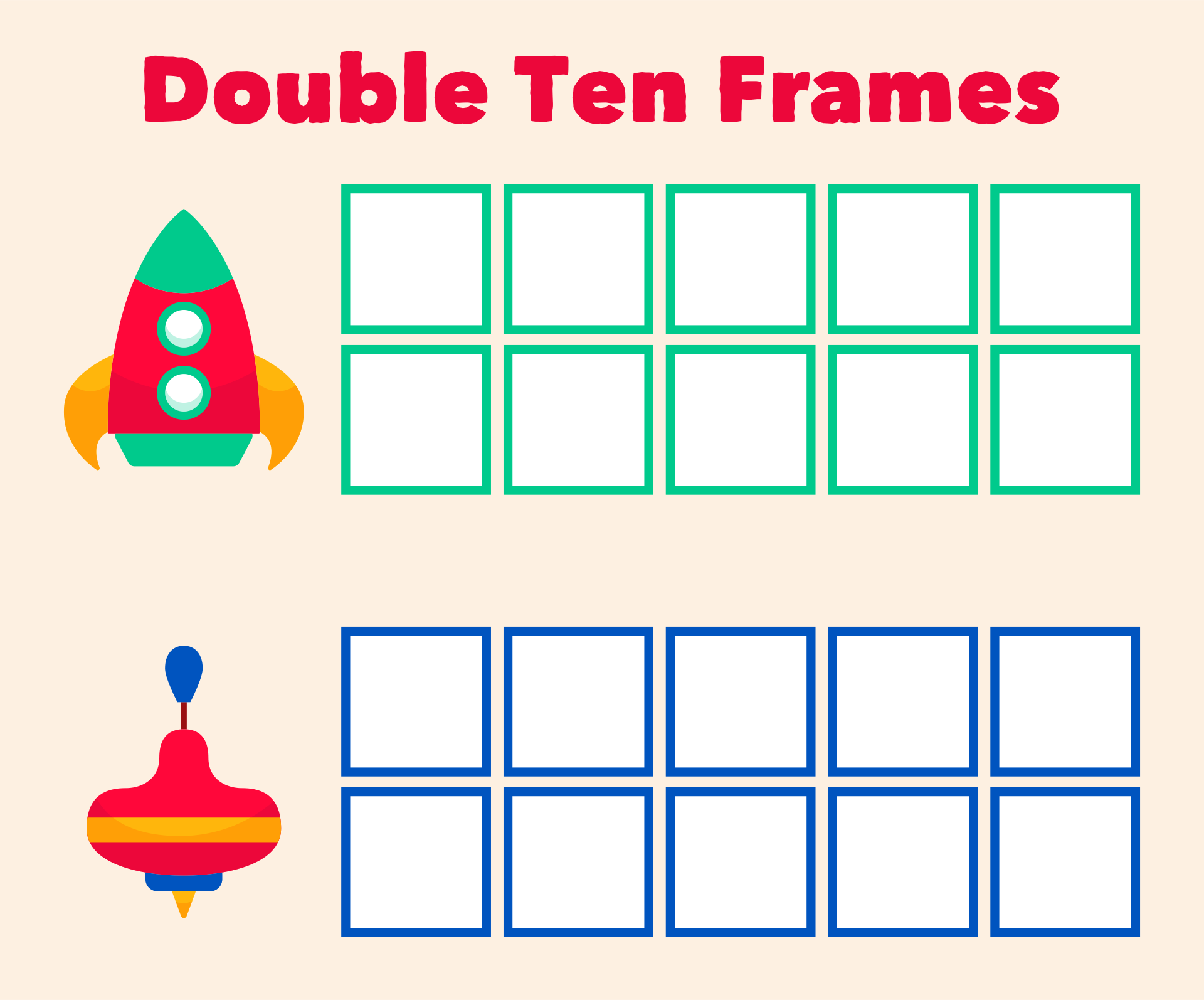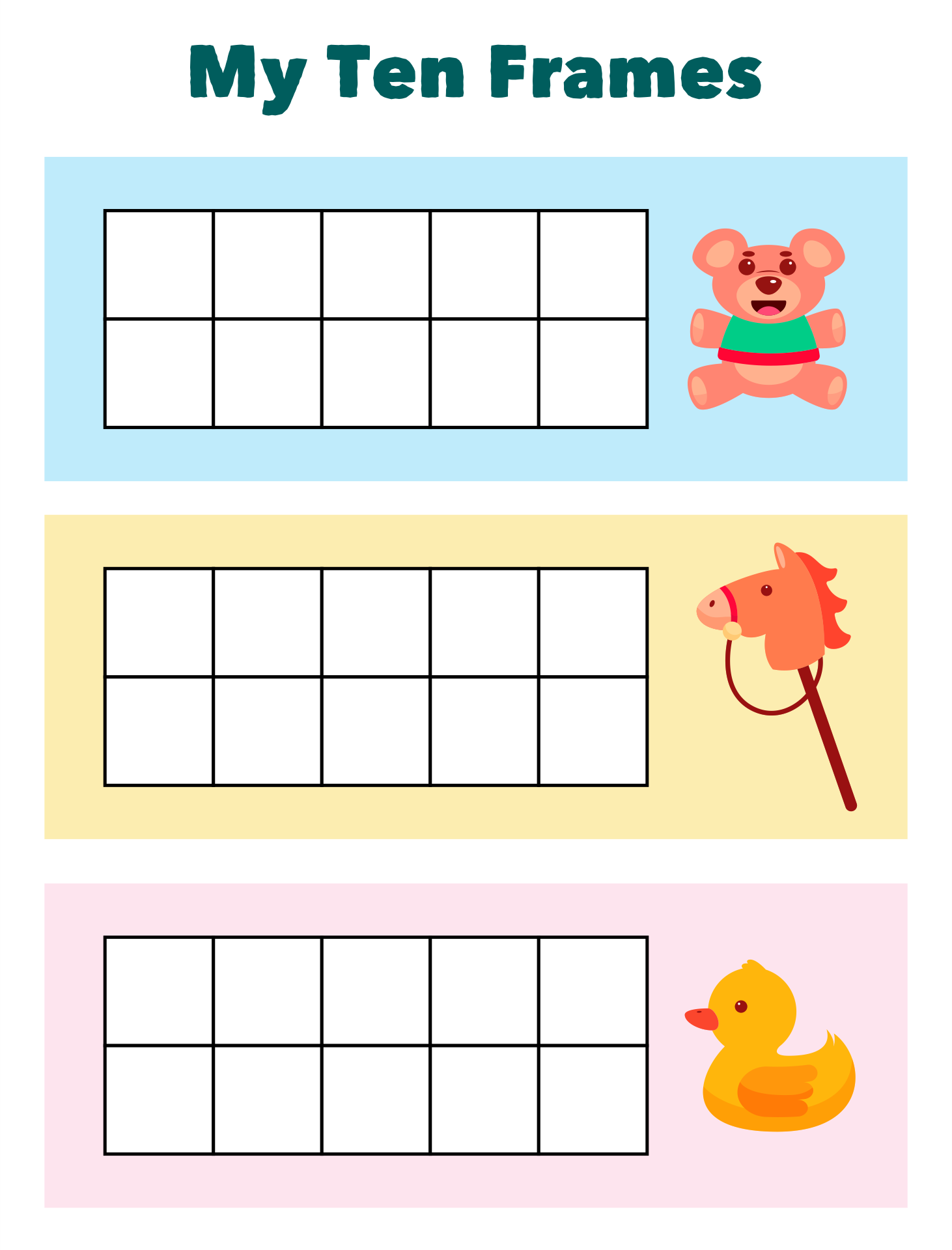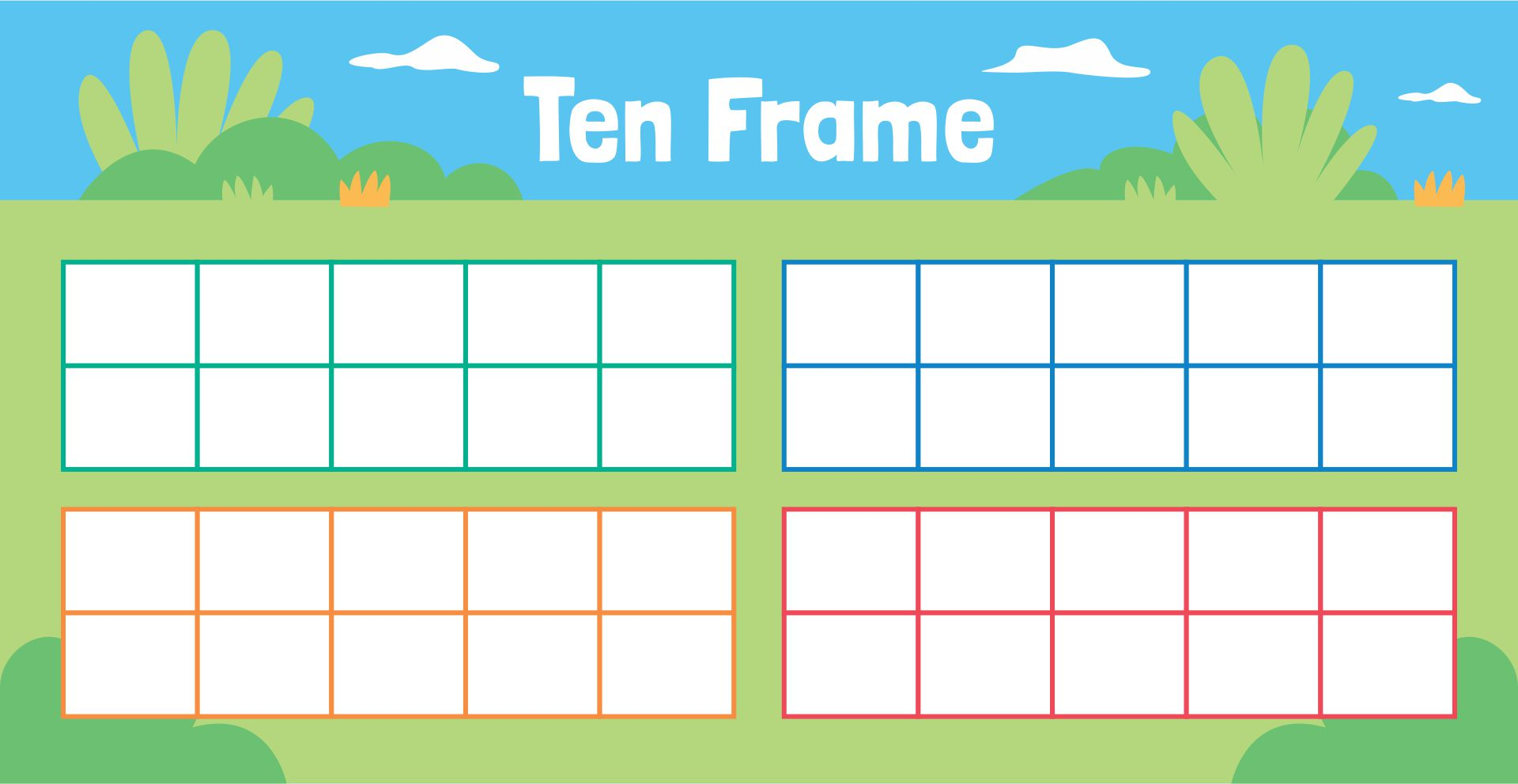### What is a good number sense?

When it comes to using ten frames, we are hoping that the number sense skills of our children will be improved. In simple words, they will get a good number sense skills after using ten frames. However, what makes the number sense skills can be identified as good?

When the number sense skills of a kid are good, they are able to manipulate numbers. They can count or calculate better and easier. The result will be more accurate. This also can increase their confidence to be able to use flexibility when it comes to solving problems.

Kids who are trained, especially the number sense skills that are trained, they can assess a reasonable answer when solving problems. They can also be familiarized with estimating answers before they count it.